Origin Of Quantum Mechanics MCQ Level – 1

# Origin Of Quantum Mechanics MCQ Level – 1

Test Description

## 10 Questions MCQ Test Topic wise Tests for IIT JAM Physics | Origin Of Quantum Mechanics MCQ Level – 1

Origin Of Quantum Mechanics MCQ Level – 1 for IIT JAM 2023 is part of Topic wise Tests for IIT JAM Physics preparation. The Origin Of Quantum Mechanics MCQ Level – 1 questions and answers have been prepared according to the IIT JAM exam syllabus.The Origin Of Quantum Mechanics MCQ Level – 1 MCQs are made for IIT JAM 2023 Exam. Find important definitions, questions, notes, meanings, examples, exercises, MCQs and online tests for Origin Of Quantum Mechanics MCQ Level – 1 below.
Solutions of Origin Of Quantum Mechanics MCQ Level – 1 questions in English are available as part of our Topic wise Tests for IIT JAM Physics for IIT JAM & Origin Of Quantum Mechanics MCQ Level – 1 solutions in Hindi for Topic wise Tests for IIT JAM Physics course. Download more important topics, notes, lectures and mock test series for IIT JAM Exam by signing up for free. Attempt Origin Of Quantum Mechanics MCQ Level – 1 | 10 questions in 30 minutes | Mock test for IIT JAM preparation | Free important questions MCQ to study Topic wise Tests for IIT JAM Physics for IIT JAM Exam | Download free PDF with solutions
 1 Crore+ students have signed up on EduRev. Have you?
Origin Of Quantum Mechanics MCQ Level – 1 - Question 1

### If first excitation potential of a hydrogen-like atom is V eV, then the ionization energy of this atom will be :

Detailed Solution for Origin Of Quantum Mechanics MCQ Level – 1 - Question 1

First excitation energy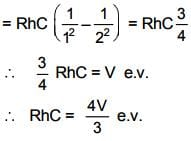Origin Of Quantum Mechanics MCQ Level – 1 - Question 2

### Linear momenta of a proton and an electron are equal. Relative to an electron

Detailed Solution for Origin Of Quantum Mechanics MCQ Level – 1 - Question 2

If pe = pp,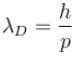Origin Of Quantum Mechanics MCQ Level – 1 - Question 3

### The wavelength of a photon and the de-Broglie wavelength of an electron and uranium atom are identical. Which one of them will have highest kinetic energy

Detailed Solution for Origin Of Quantum Mechanics MCQ Level – 1 - Question 3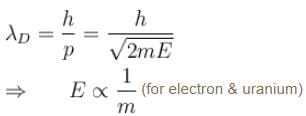for photon & electron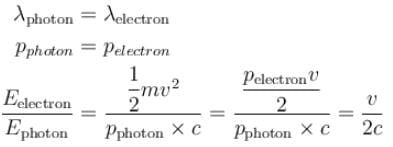Origin Of Quantum Mechanics MCQ Level – 1 - Question 4

The energy E and momentum p of photon is given by E = hv and  p = h/λ, the velocity of photon will be :

Detailed Solution for Origin Of Quantum Mechanics MCQ Level – 1 - Question 4

E = hc/λ     put c = v
and  p = h/λ
E = pv
⇒ v = E/p

Origin Of Quantum Mechanics MCQ Level – 1 - Question 5

In the figure six lines of emission spectrum are shown. Which of them will be absent in the absorption spectrum.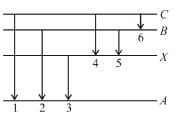Detailed Solution for Origin Of Quantum Mechanics MCQ Level – 1 - Question 5

The photon’s with energies equal to that required for upward transition A → X, A → B and A → C would be absorbed, these photons shall be absent from absorption spectrum.

The correct answer is: 1, 2, 3

Origin Of Quantum Mechanics MCQ Level – 1 - Question 6

A charge particle q0 of mass m0 is projected along the y-axis at t = 0 from origin with a velocity V0. If uniform electric field E0 also exists along the x-axis, then the time at which de-Broglie wavelength of the particle becomes half of the initial value is :

Detailed Solution for Origin Of Quantum Mechanics MCQ Level – 1 - Question 6

Initial debrogle wavelength of the charge particle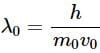After any time t,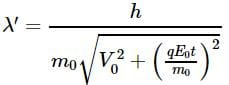Suppose after time t,  λ becomes half of the initial value i.e.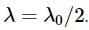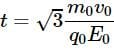Origin Of Quantum Mechanics MCQ Level – 1 - Question 7

What voltage must be applied to an electron microscope to produce electrons of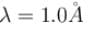Detailed Solution for Origin Of Quantum Mechanics MCQ Level – 1 - Question 7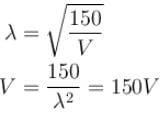The correct answer is: 150 Volt

Origin Of Quantum Mechanics MCQ Level – 1 - Question 8

In X-rays production an electron accelerated with voltage V strikes a metal target. For which of the following voltages X-rays of minimum wavelength will be produced :

Detailed Solution for Origin Of Quantum Mechanics MCQ Level – 1 - Question 8

λ will be minimum for maximum accelerated voltage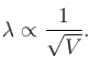The correct answer is: 40 kV

Origin Of Quantum Mechanics MCQ Level – 1 - Question 9

In the photoelectric, if we use a monochromatic light, the I-V curve is as shown. If work function of the metal is 2eV, estimate the power of light used. (Assume efficiency of photo emission = 10–3 % i.e. number of photoelectrons emitted are 10–3 % of number of photons incident on metal.)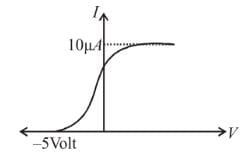Detailed Solution for Origin Of Quantum Mechanics MCQ Level – 1 - Question 9

The energy of incident photons is given by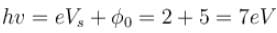(Vs is stopping potential and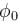is work function)
Saturation current =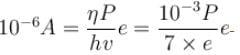(η is photo emission efficiency)
∴ P = 7mW

Origin Of Quantum Mechanics MCQ Level – 1 - Question 10

In Davisson-Germer experiment the relation between the angle of diffraction θ and the grazing angle φ is.

Detailed Solution for Origin Of Quantum Mechanics MCQ Level – 1 - Question 10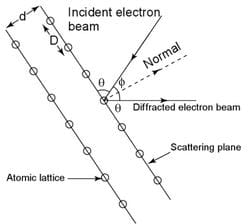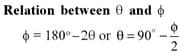## Topic wise Tests for IIT JAM Physics

217 tests
Information about Origin Of Quantum Mechanics MCQ Level – 1 Page
In this test you can find the Exam questions for Origin Of Quantum Mechanics MCQ Level – 1 solved & explained in the simplest way possible. Besides giving Questions and answers for Origin Of Quantum Mechanics MCQ Level – 1, EduRev gives you an ample number of Online tests for practice

## Topic wise Tests for IIT JAM Physics

217 tests(Scan QR code)